## Worked examples

#### Core Maths papers 1-4

OCR GCE Core Maths 1 (4721): questions 5, 7;
OCR GCE Core Maths 2 (4722): question 6;
OCR GCE Core Maths 3 (4723): questions 1, 4, 5, 6, 8;
OCR GCE Core Maths 4 (4724): questions 1, 2, 3, 5, 6, 7, 8;

Questions by kind permission OCR.
All other content © copyright ballooncalculus.org 2008.

#### Miscellaneous

Balloon Calculus
around the web...

##### Differentiate a product or quotient
√(7x - 2) / √(5x + 3) (4x + 3)7 / (5x - 2)7 3x e2x

exy

cos4(3t) sin(3t)

x(1 - x)(3/5)

3x/(x2 + 4)

x2 e3x2

(4 - x2) / [ (x2 + 4) ]2

(1-s2 ) / (1 + s2 )2

(12x3 + 3x - 6) (3x-2 + 5x-3)

(2x2 + 3)4 (3x - 1)5

##### Differentiate a composite function
[(4x + 3) / (5x - 2)]7 esec x

(4 - 2x2)6

ln | x2 + 5 |

(6x4 - 6x -3 - 2x + 5) -2

- ln | cos x |

ln | 8x3 - 7x + 2.78 |

##### Implicit differentiation
2x2y = sin(2x)

x2y = x + y2

sin3x cos2y = 6 3x2 + 4xy3 = 9 6x + 10y dy/dx - 6 + 20 dy/dx

x2 - xy + ¾ x2 = 7

##### Change of variable
∫ 8(y - 9)-3 dy ∫ 3x e2x2 - 1 dx

e- x dx

∫ sec2x / (4 + tan2x) dx

∫ arctan x / (1 + x2) dx

∫ sec x tan x dx

∫ 1 / [(a - x)(b - x)] dx

∫ ln | 1 - x | dx

∫ 2x / √(9 - 4x4) dx

∫ sin2u) dx

x2 / (2 + x6) dx

∫ cos(a - x) dx

∫ tan5x sec4x dx

∫ cos4(3t) sin(3t) dt

∫ sin x cos ( cos x ) dx

0π / 3 sin θ / cos2 θ dθ

∫ 4t / (1 + 16t2) dt

∫ - 4x / (2x2 + 1)2 dx

∫ 6 sec2(5x) / [7 - 3 tan(5x)]7 dx

∫ ½ ex / √x dx

13 (x + 3) / (x2 + 6x) dx

0π sin t / (1 + cos2 t) dt

∫ cos3x dx

∫ 4x / (x2 + 3) dx

y √(1 - y) dy

∫ e2x (1 + e2x)3 dx

x3 (x4 + 3)2 dx

##### Internal substitution
∫ 1 / √(1 - x2) dx

∫ 1/√(x2 - 9/4) dx

##### Weierstrass substitution
∫ 2 / (2 cosx + sinx) dx

∫ secx dx

∫ cosecx dx

##### Parts
∫ √(a2 - x2) dx ∫ (x + 2) (1 - e-x/3) dx ∫ 5x e4x dx

∫ x e-x/4 dx

∫ √( 2 + t2 ) dt

∫ ln (x2 - x + 2) dx

∫ ln (x + 1) dx

∫ ln (2x + 1) dx

∫ ln x dx

##### Parts twice
x2 cos (π x) dx

ex sin(2x) dx

x2 cos x dx

x2 sin(3x + 1) dx

x3 sin x dx

##### Separation of variables
dh/dt = (6 - h) /20

dy/dx = Cy

##### Integrating factor
dy/dx - y / (x + 1) = x - 1

dy/dx + 3x2y = 6x2

##### Notation
dv and v'

d/dx f -1(x)

In Balloon Calculus, we use three shapes: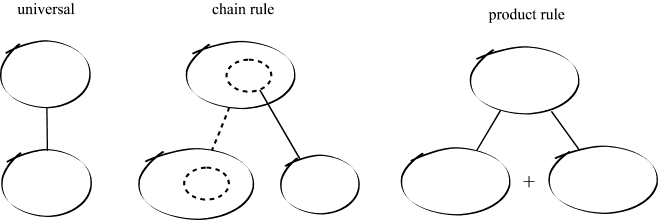Use the examples on this page to see how these shapes can ease up the trickier bits of calculus working. They work on two basic assumptions:

A continuous straight line differentiates going down, integrates going up. The dashed/dotted straight line (in the chain-rule shape) is similar, except that the differentiation or integration is 'with respect to' the expression in the dashed/dotted balloon. The product-rule shape is different, in that the two lines in the zoomed-out version of this shape don't operate independently, but only so as to emphasise the second assumption...

In any shape, the whole of the lower level is the derivative of the whole of the top (i.e. the top is integral of the bottom).

Common sense? Let us know if you agree!

### OCR AS-level Core Maths 1 June 2007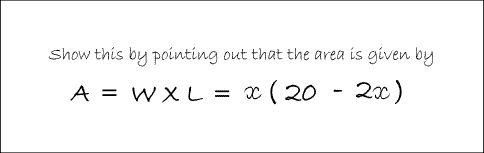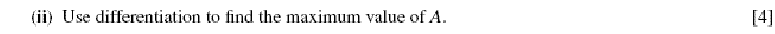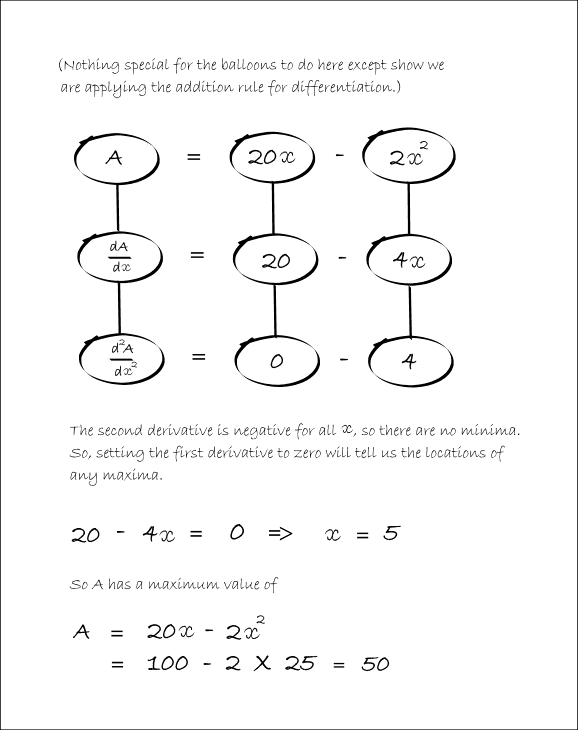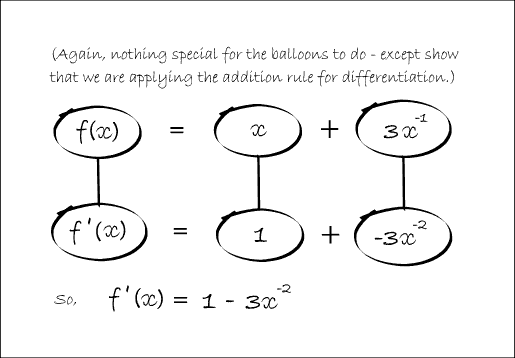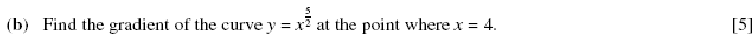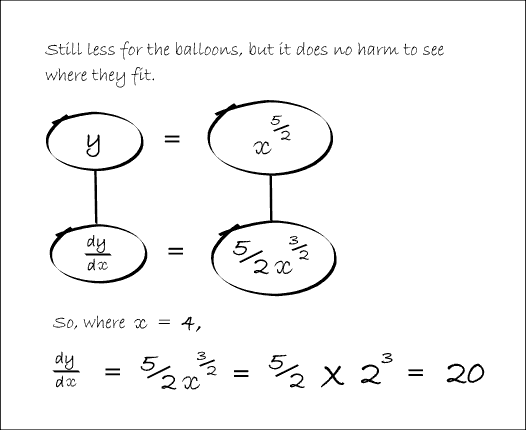### OCR AS-level Core Maths 2 June 2007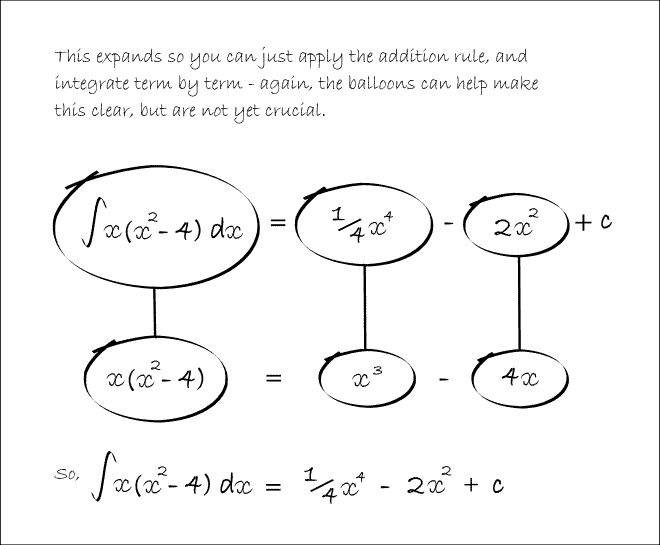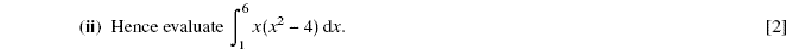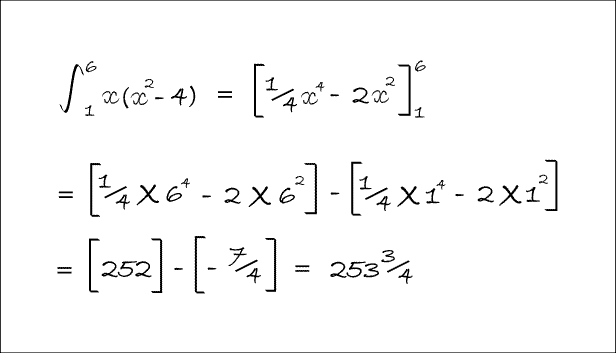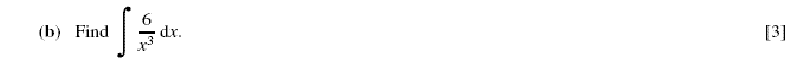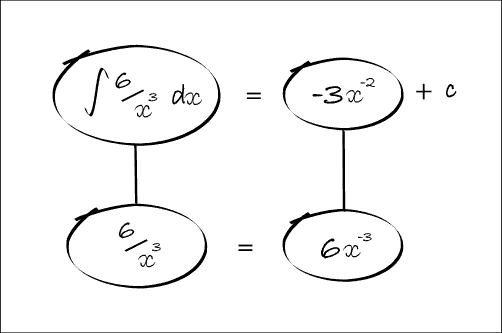### OCR AS-level Core Maths 3 June 2007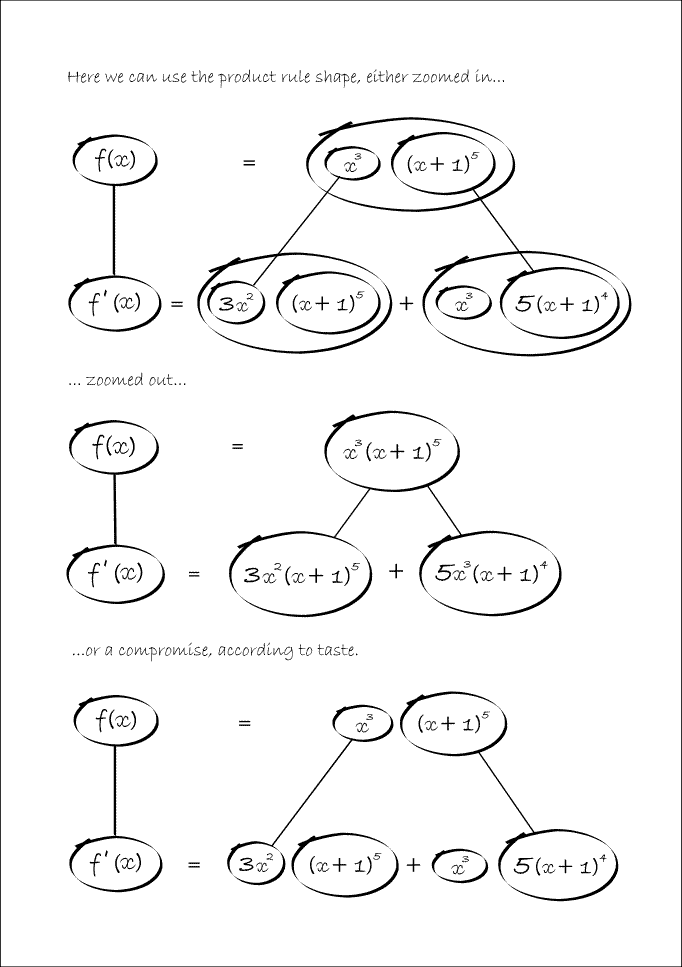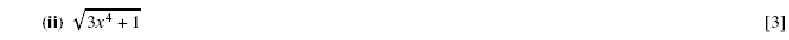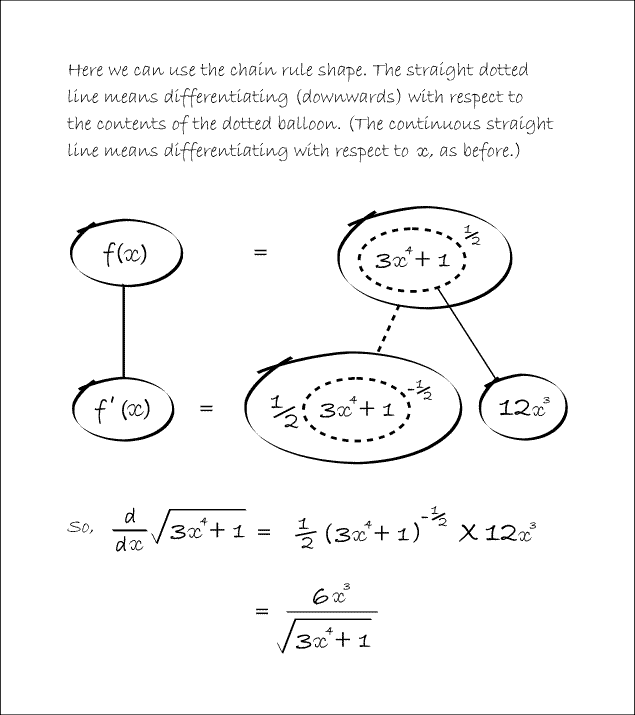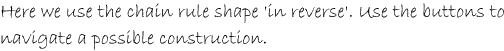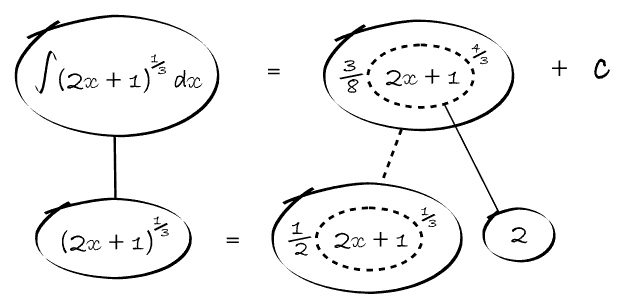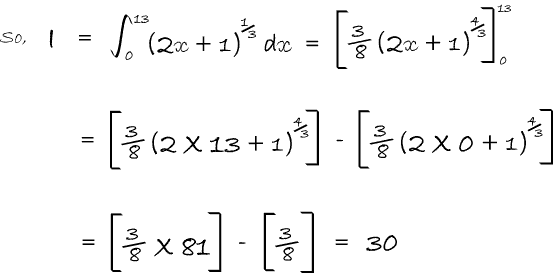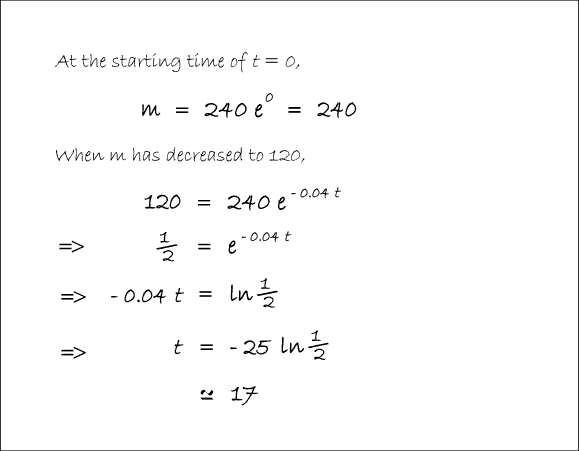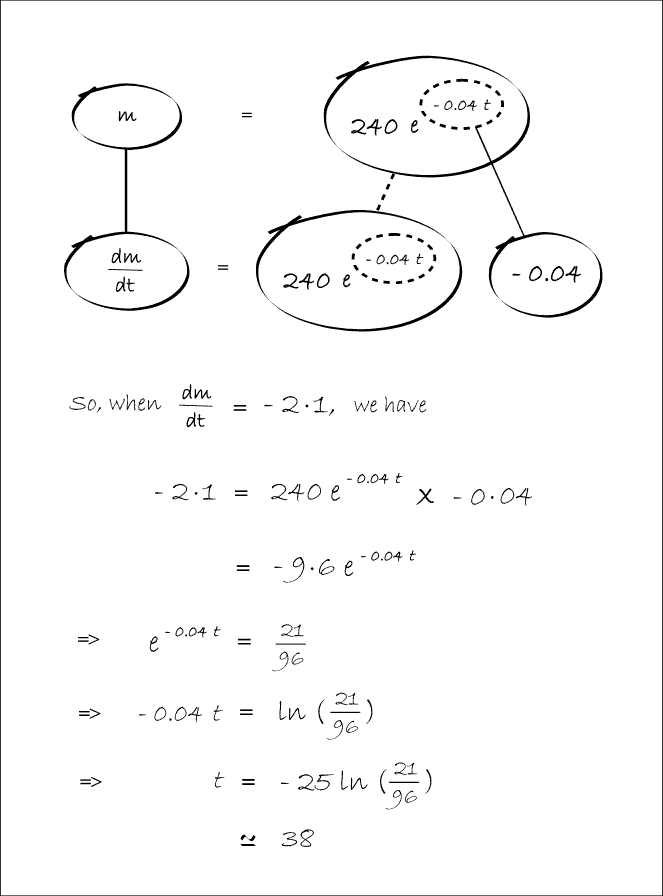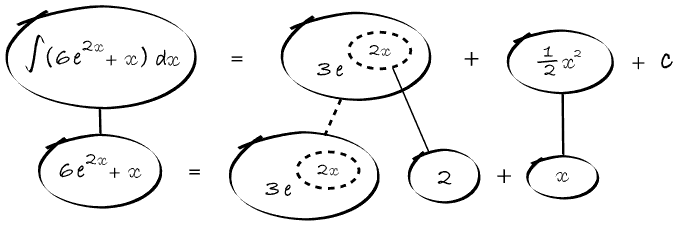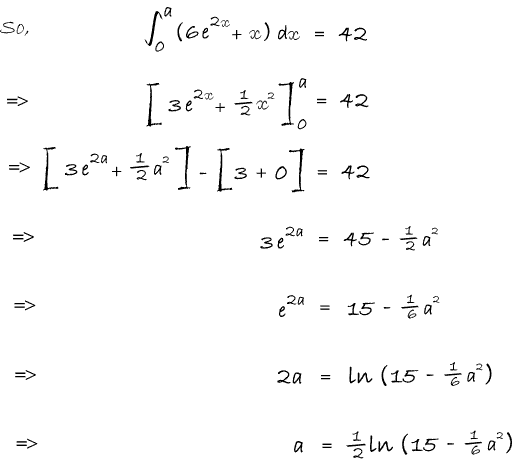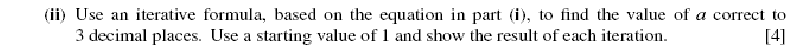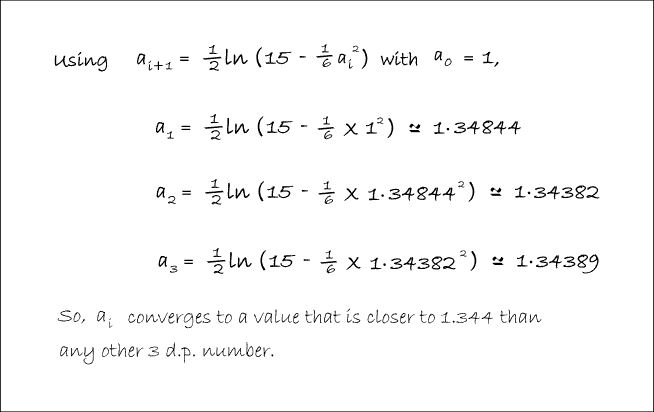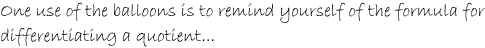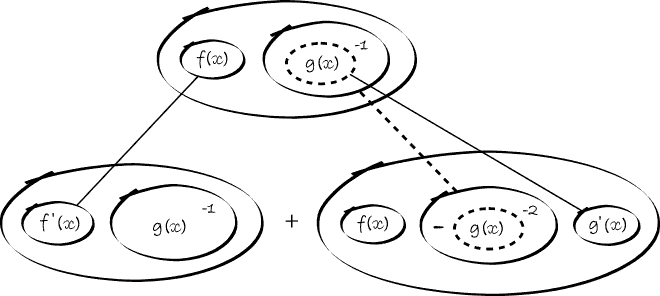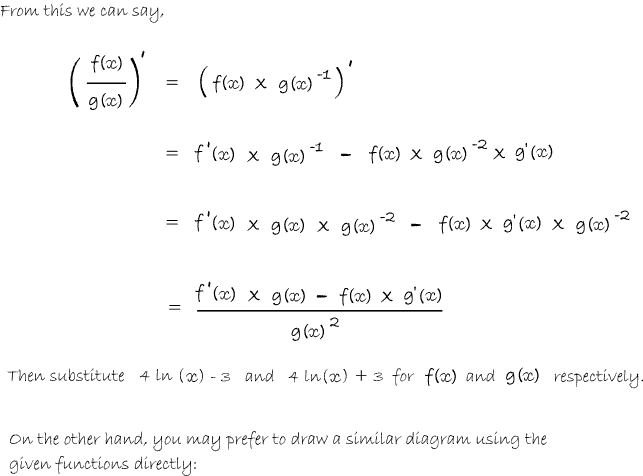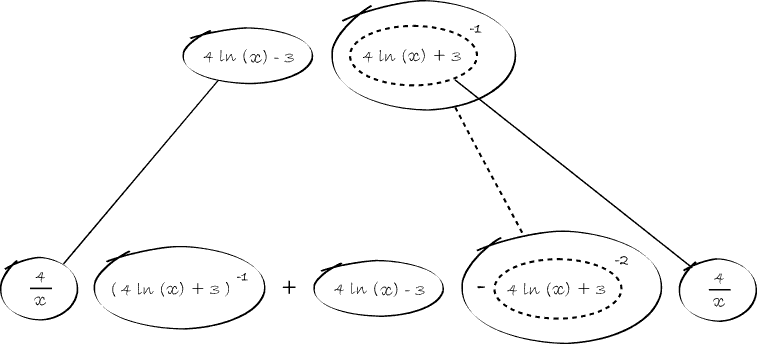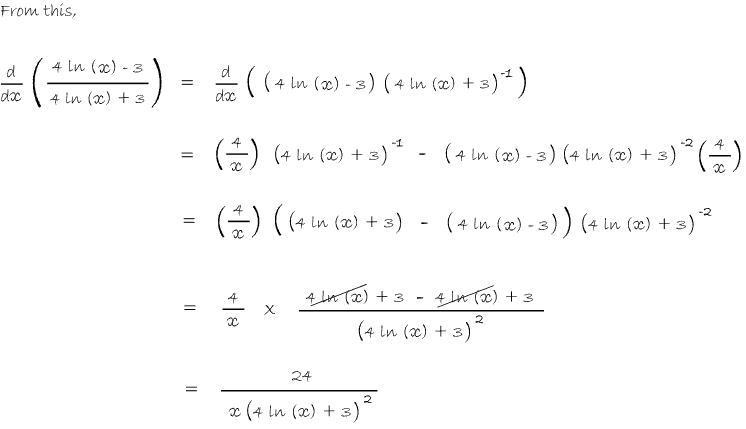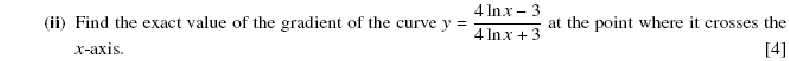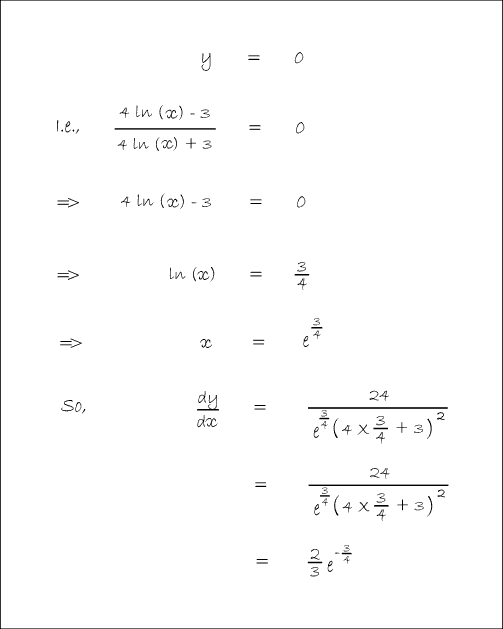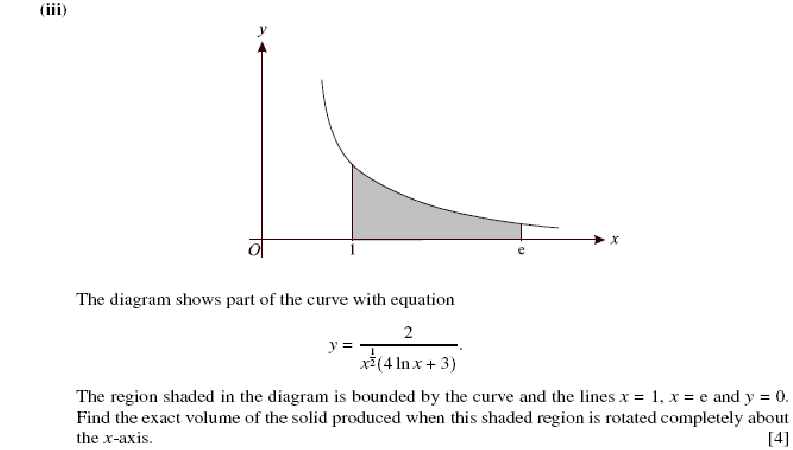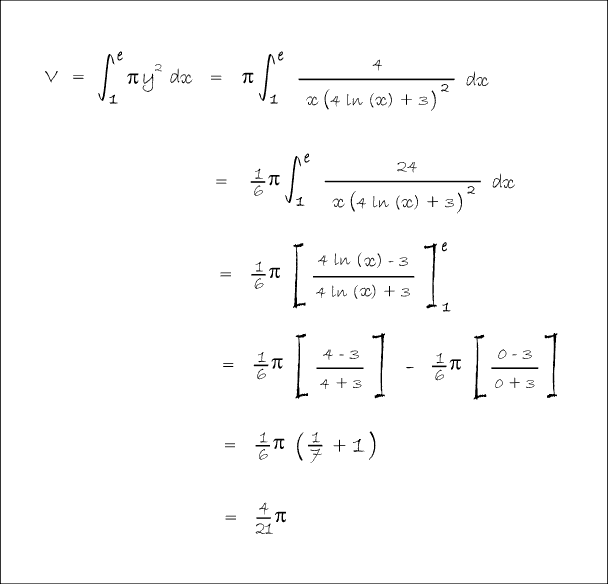### OCR AS-level Core Maths 4 June 2007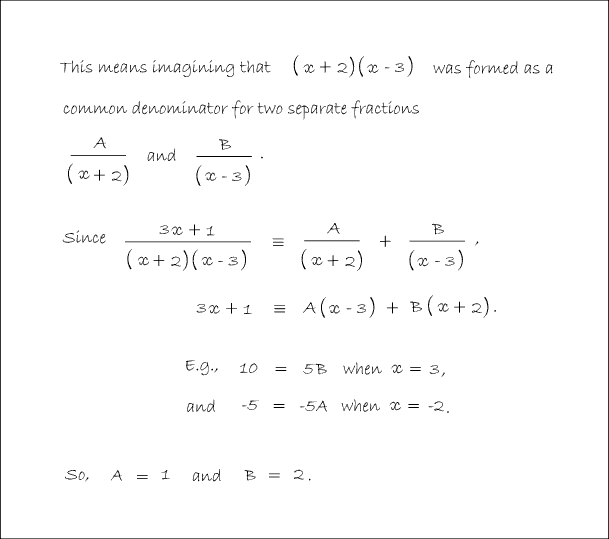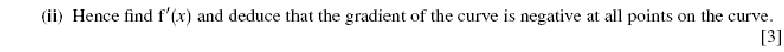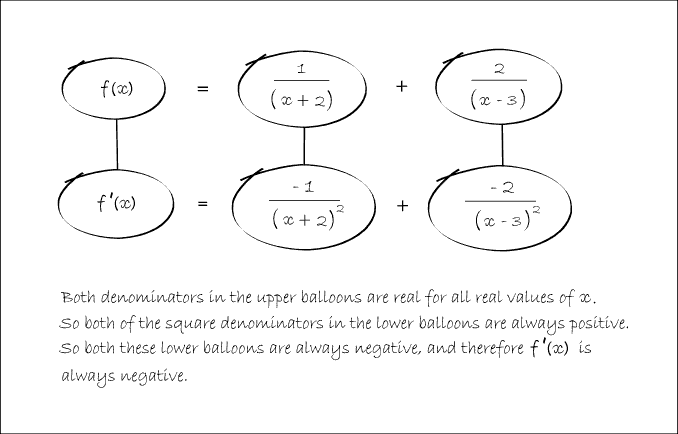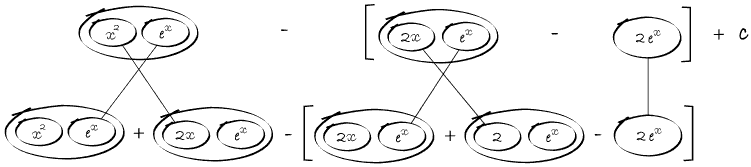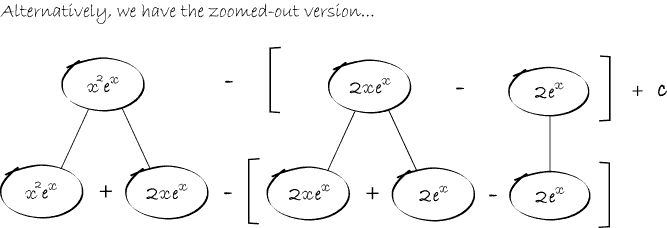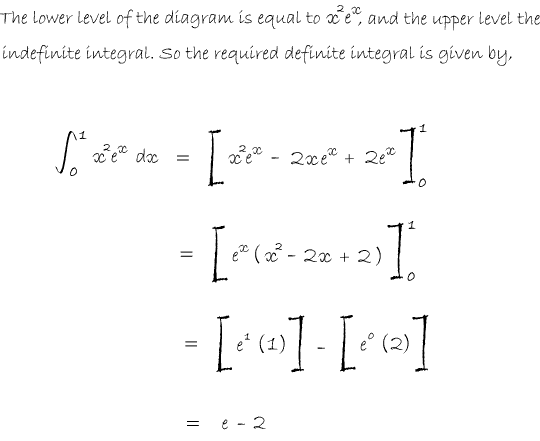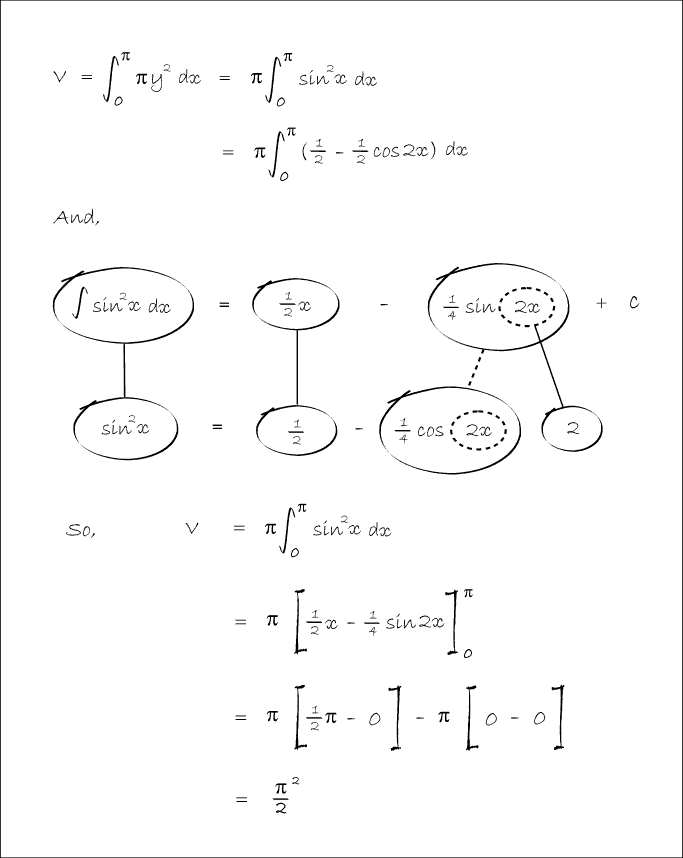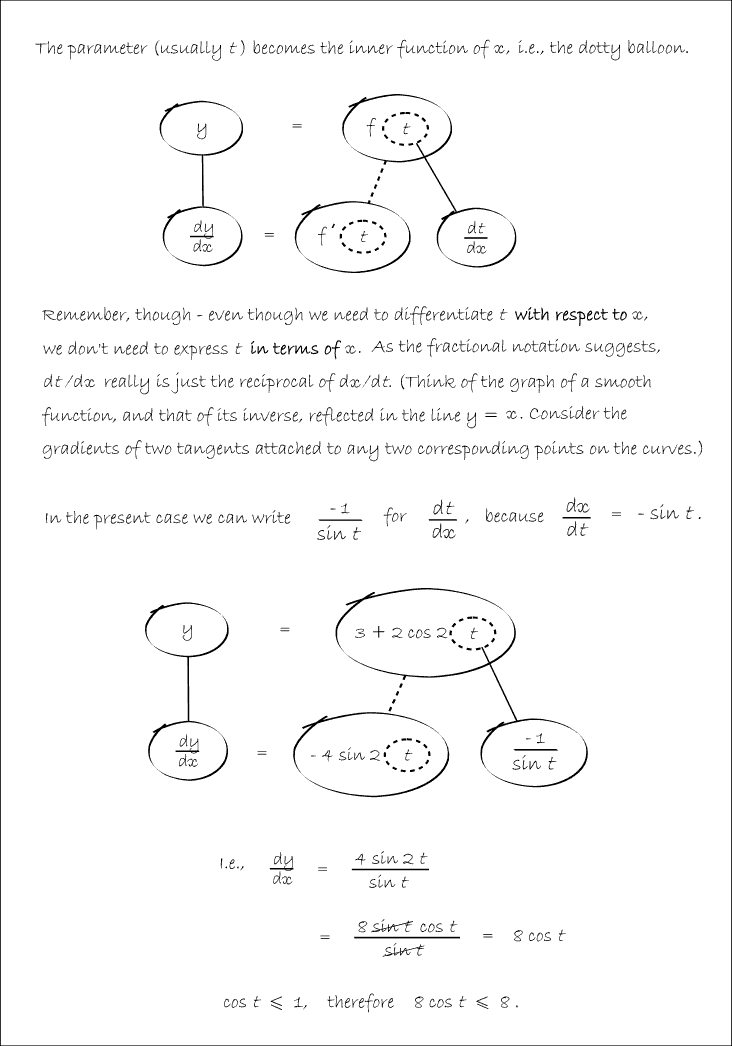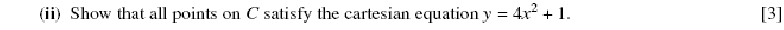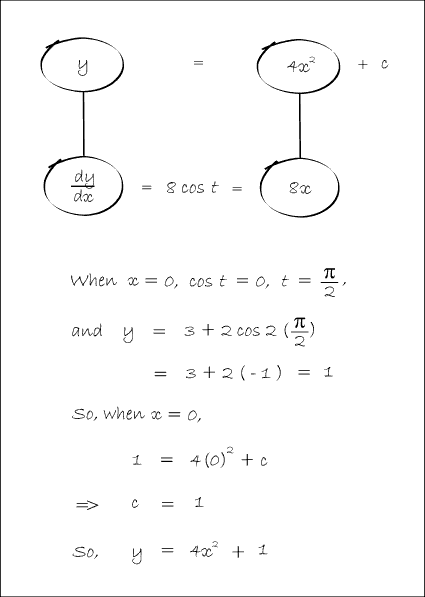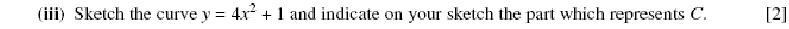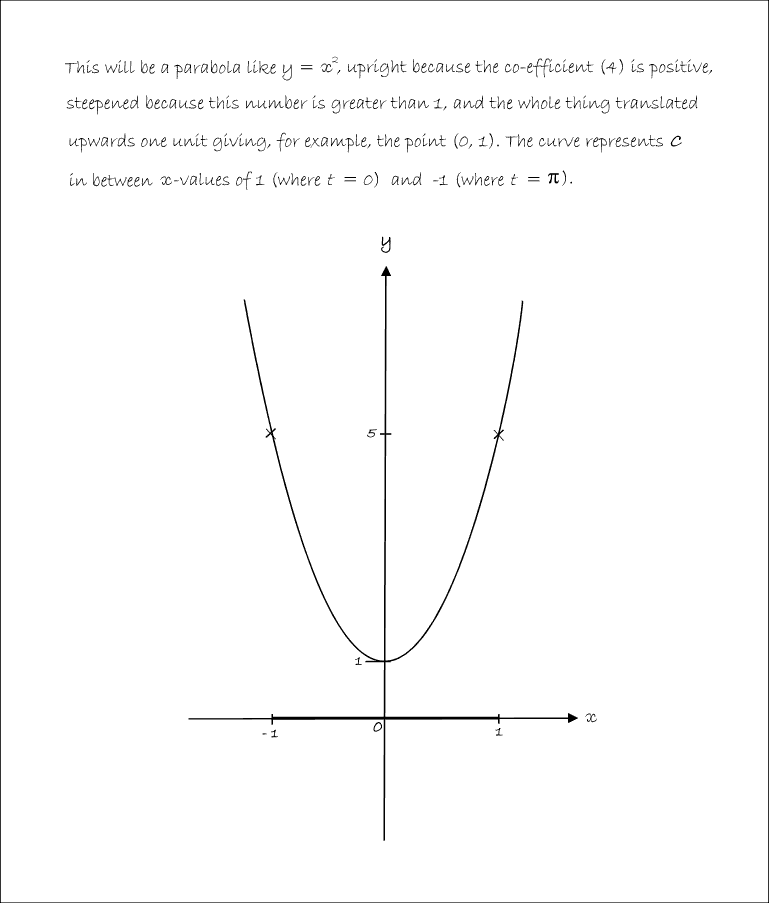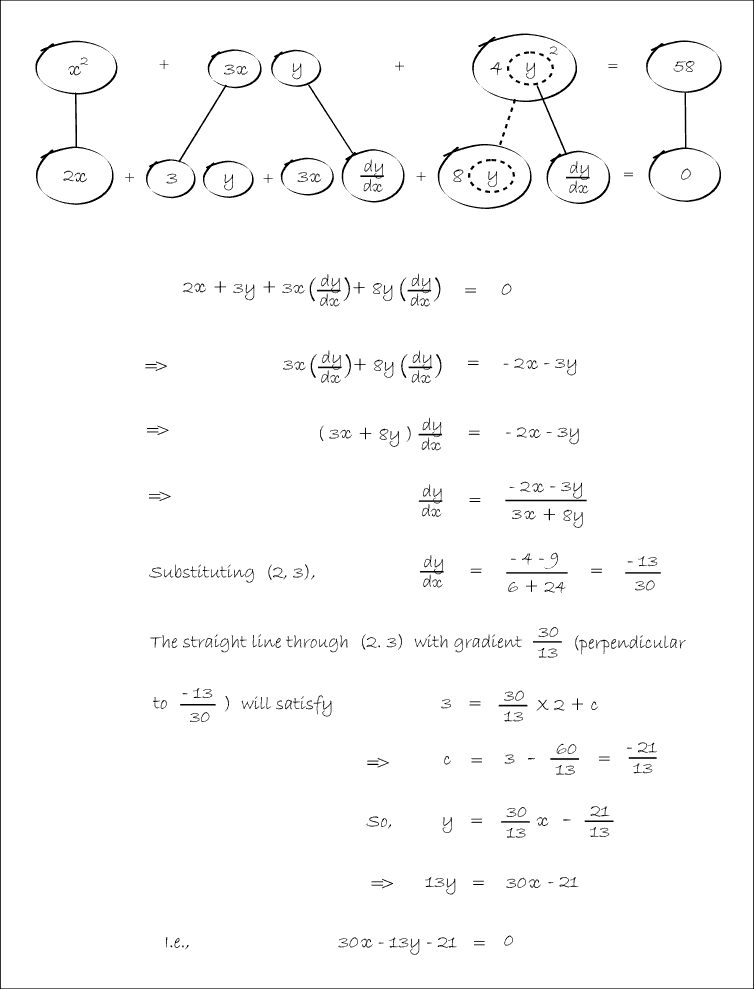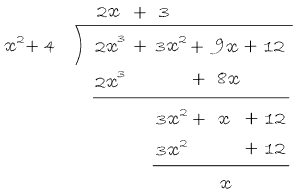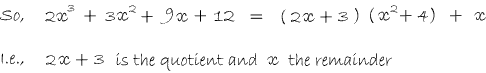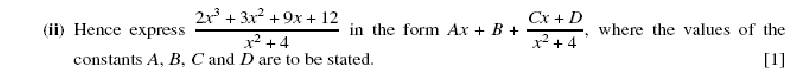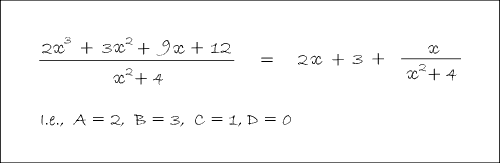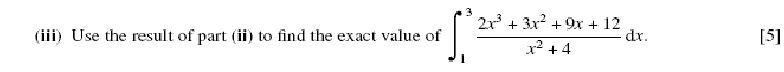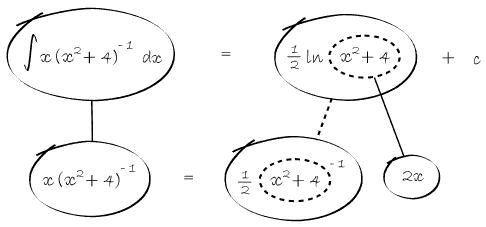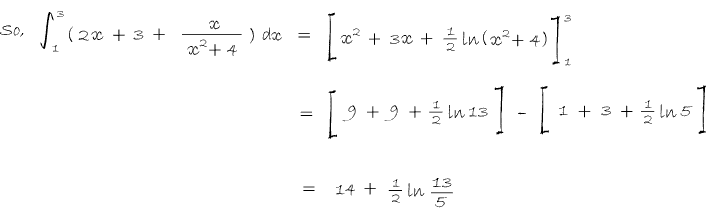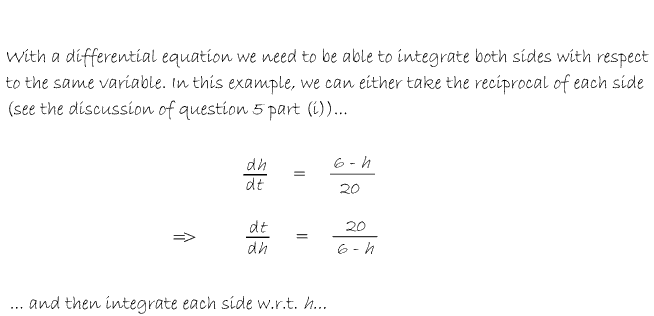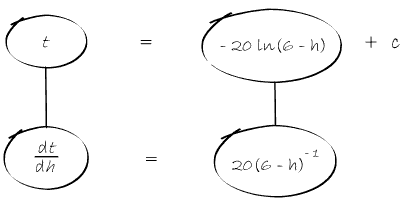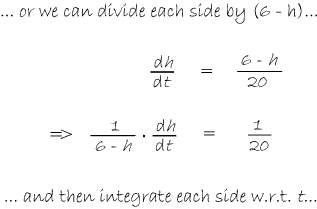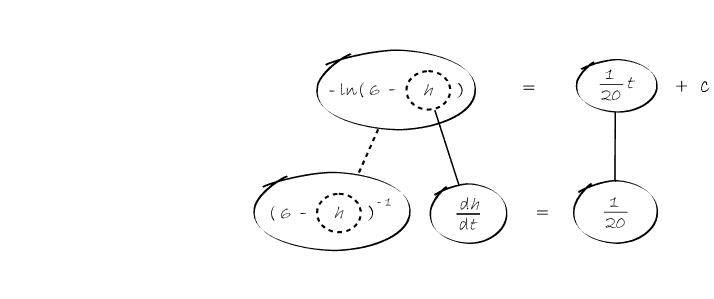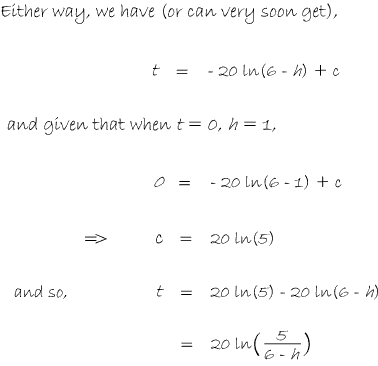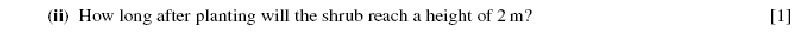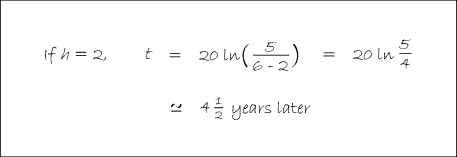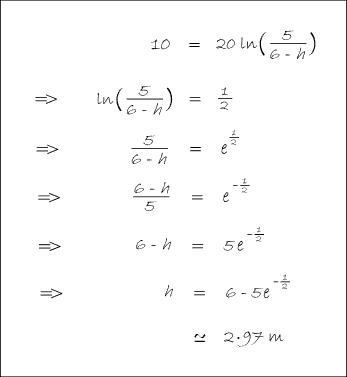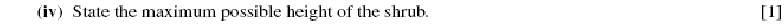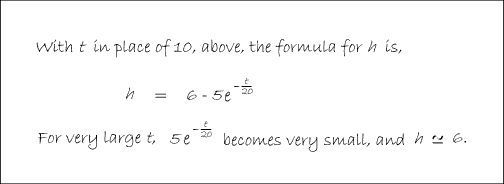© Copyright ballooncalculus.org 2007 - 2009. All rights reserved. Email: tom@ballooncalculus.org